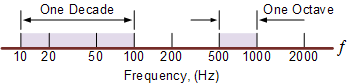# Butterworth Filter Design

## Butterworth Filter Design

In the previous filter tutorials we looked at simple first-order type low and high pass filters that contain only one single resistor and a single reactive component (a capacitor) within their RC filter circuit design.In applications that use filters to shape the frequency spectrum of a signal such as in communications or control systems, the shape or width of the roll-off also called the “transition band”, for a simple first-order filter may be too long or wide and so active filters designed with more than one “order” are required. These types of filters are commonly known as “High-order” or “nth-order” filters.

The complexity or filter type is defined by the filters “order”, and which is dependant upon the number of reactive components such as capacitors or inductors within its design. We also know that the rate of roll-off and therefore the width of the transition band, depends upon the order number of the filter and that for a simple first-order filter it has a standard roll-off rate of 20dB/decade or 6dB/octave.

Then, for a filter that has an nth number order, it will have a subsequent roll-off rate of 20n dB/decade or 6n dB/octave. So a first-order filter has a roll-off rate of 20dB/decade (6dB/octave), a second-order filter has a roll-off rate of 40dB/decade (12dB/octave), and a fourth-order filter has a roll-off rate of 80dB/decade (24dB/octave), etc, etc.

High-order filters, such as third, fourth, and fifth-order are usually formed by cascading together single first-order and second-order filters.

For example, two second-order low pass filters can be cascaded together to produce a fourth-order low pass filter, and so on. Although there is no limit to the order of the filter that can be formed, as the order increases so does its size and cost, also its accuracy declines.

One final comment about Decades and Octaves. On the frequency scale, a Decade is a tenfold increase (multiply by 10) or tenfold decrease (divide by 10). For example, 2 to 20Hz represents one decade, whereas 50 to 5000Hz represents two decades (50 to 500Hz and then 500 to 5000Hz).

An Octave is a doubling (multiply by 2) or halving (divide by 2) of the frequency scale. For example, 10 to 20Hz represents one octave, while 2 to 16Hz is three octaves (2 to 4, 4 to 8 and finally 8 to 16Hz) doubling the frequency each time. Either way, Logarithmic scales are used extensively in the frequency domain to denote a frequency value when working with amplifiers and filters so it is important to understand them.

### Logarithmic Frequency ScaleSince the frequency determining resistors are all equal, and as are the frequency determining capacitors, the cut-off or corner frequency ( ƒC ) for either a first, second, third or even a fourth-order filter must also be equal and is found by using our now old familiar equation:As with the first and second-order filters, the third and fourth-order high pass filters are formed by simply interchanging the positions of the frequency determining components (resistors and capacitors) in the equivalent low pass filter. High-order filters can be designed by following the procedures we saw previously in the Low Pass and High Pass filter tutorials. However, the overall gain of high-order filters is fixed because all the frequency determining components are equal.

## Filter Approximations

So far we have looked at a low and high pass first-order filter circuits, their resultant frequency and phase responses. An ideal filter would give us specifications of maximum pass band gain and flatness, minimum stop band attenuation and also a very steep pass band to stop band roll-off (the transition band) and it is therefore apparent that a large number of network responses would satisfy these requirements.